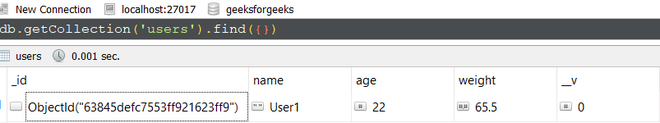Open in App
Not now

# Mongoose Schematype.get() API

• Last Updated : 05 Dec, 2022

Mongoose is a MongoDB object modeling and handling for a node.js environment. The Schematype.get() method of the Mongoose API is used to get the manipulate or transform data from MongoDB database or Schema. Using this function we can modify the value of any attribute in the database based on our requirement. Let us understand Mongoose Schematype.get() method using example.

Syntax:

`SchemaType.get(<function>)`

Parameters: The Schematype.get() method accepts a single parameter:

• getterFunction: It is the function that return the modified or manipulated data.

Return Value: The Schematype.get() function returns access to all the instances of this schema type.

Setting up Node.js application:

Step 1: Create a Node.js application using the following command:

`npm init`

Step 2: After creating the NodeJS application, Install the required module using the following command:

`npm install mongoose`

Project Structure: The project structure will look like this:Database Structure: The database structure will look like this, the following documents are present in the collection.Example 1: In this example, We have established a database connection using mongoose and defined model over userSchema, having three columns or fields “name”, “age”, and “weight”. At the schema level on “weight” attribute we have defined getter function that will return round up value of “weight”.

Filename: app.js

## Javascript

 `// Require mongoose module ` `const mongoose = require(``"mongoose"``); ` ` `  `// Set Up the Database connection ` `mongoose.connect(``"mongodb://localhost:27017/geeksforgeeks"``, { ` `    ``useNewUrlParser: ``true``, ` `    ``useUnifiedTopology: ``true``, ` `}); ` ` `  `// Define a user schema ` `const userSchema = ``new` `mongoose.Schema({ ` `    ``name: { ` `        ``type: String ` `    ``}, ` `    ``age: { ` `        ``type: Number ` `    ``}, ` `    ``weight: { ` `        ``type: Number, ` `        ``get: (value) => { ` `            ``return` `Math.ceil(value) ` `        ``} ` `    ``} ` `}); ` ` `  `const User = mongoose.model(``'User'``, userSchema); ` ` `  `// Find the user by given ID ` `User.findById(``'63845defc7553ff921623ff9'``).then(result => { ` `    ``console.log(result.weight); ` `});`

Step to run the program: To run the application execute the below command from the root directory of the project:

`node app.js`

Output:

`66`

Example 2: In this example, We have established a database connection using mongoose and defined a model over userSchema, having four columns or fields “name”, “age”, “weight”, and “height”. At the schema level on the “height” attribute, we have defined getter function that will return height in centimeter.

Filename: app.js

## Javascript

 `// Require mongoose module ` `const mongoose = require(``"mongoose"``); ` ` `  `// Set Up the Database connection ` `mongoose.connect(``"mongodb://localhost:27017/geeksforgeeks"``, { ` `    ``useNewUrlParser: ``true``, ` `    ``useUnifiedTopology: ``true``, ` `}); ` ` `  `// Define the user schema ` `const userSchema = ``new` `mongoose.Schema({ ` `    ``name: { ` `        ``type: String ` `    ``}, ` `    ``age: { ` `        ``type: Number ` `    ``}, ` `    ``weight: { ` `        ``type: Number, ` `        ``get: (value) => { ` `            ``return` `Math.ceil(value) ` `        ``} ` `    ``}, ` `    ``height: { ` `        ``type: Number, ` ` `  `        ``// Define the getter function ` `        ``get: (value) => { ` `            ``return` `value * 30.48 ` `        ``} ` `    ``} ` `}); ` ` `  `const User = mongoose.model(``'User'``, userSchema); ` ` `  `const user = ``new` `User({  ` `    ``name: ``"User1"``, age: 25, ` `    ``weight: 68.5, height: 5.8 ` `}) ` `user.save() ` `console.log(``'Height in cm -'``, user.height)`

Step to run the program: To run the application execute the below command from the root directory of the project:

`node app.js`

Output:

`Height in cm - 176.784`

My Personal Notes arrow_drop_up
Related Articles